# Subtraction Facts to 10

Subtraction Facts to 10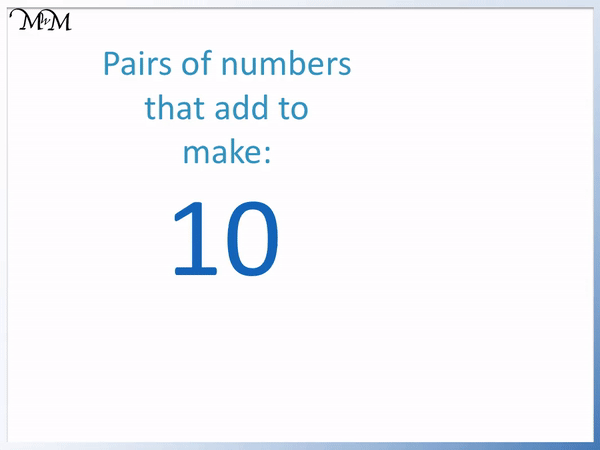• The pairs of numbers which add to make 10 are 1 + 9, 2 + 8, 3 + 7, 4 + 6 and 5 + 5.
• We can use these fact families to learn subtractions from 10.• Because this is a subtraction from 10, we can use our pairs of numbers that add to make 10.
• We are subtracting 6 from 10 and so, we look at which number pairs with 6 to make 10.
• 4 + 6 = 10 and therefore, 10 – 6 = 4.

You can solve subtraction facts using number bonds that add to make the number that you are subtracting from.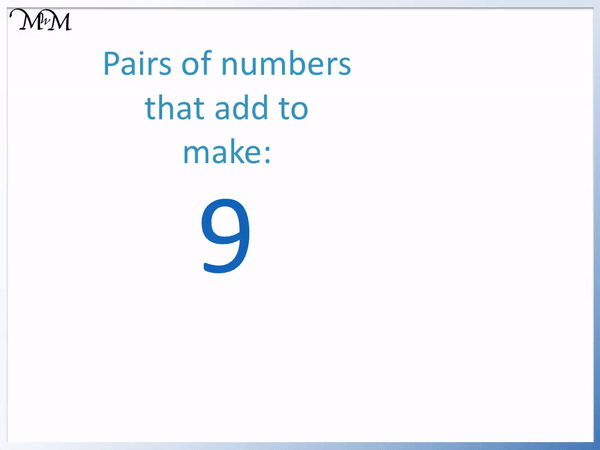• To solve a subtraction from 9, it is helpful to know the pairs of numbers that add to make 9.
• The numbers that add to make 9 are 1 + 8, 2 + 7, 3 + 6 and 4 + 5.• This is a subtraction from 9 and so, we can use the pairs of numbers that add to make 9.
• Subtracting something from 9 equals 3.
• This number will be the same number that can be added to 3 to make 9.
• 3 + 6 = 9 and so, we add 6 to 3 to make 9.
• Therefore 9 – 6 = 3.# What are Subtraction Facts?

Subtraction facts are combinations of numbers written as subtraction sums involving a subtraction sign ‘-‘ and an equals sign ‘=’.

For example, 10 – 5 = 5 is a subtraction fact. It is a subtraction fact from 10, since 10 is the number that is being subtracted from.Simple subtraction facts are taught in school alongside addition facts. It is useful to memorise the subtraction facts up to 10 because they will help when subtracting all larger numbers mentally.

To learn subtraction facts, it is easiest to learn the pairs of numbers that add to make the number that is being subtracted from.

In this lesson we will look at subtraction facts to 10, 9, 8 and 7.

## Subtraction Facts to 10

Subtraction facts to 10 are subtractions from 10 that should be learnt. 10 will be the first number in the equation and it will be followed by a subtraction sign.

To learn the subtraction facts to 10, you need to know your number bonds to 10.

These are the pairs of numbers that add to make 10.Here is the full list of number bonds to 10.

We can see that as the first number in each pair increases from 1 to 2, 2 to 3, 3 to 4 and 4 to 5, the second number decreases by one each time from 9 to 8, 8 to 7, 7 to 6 and 6 to 5.

When teaching subtraction facts to 10, it is important to show these number bonds and to look for this simple pattern. There are only 5 pairs of numbers to remember.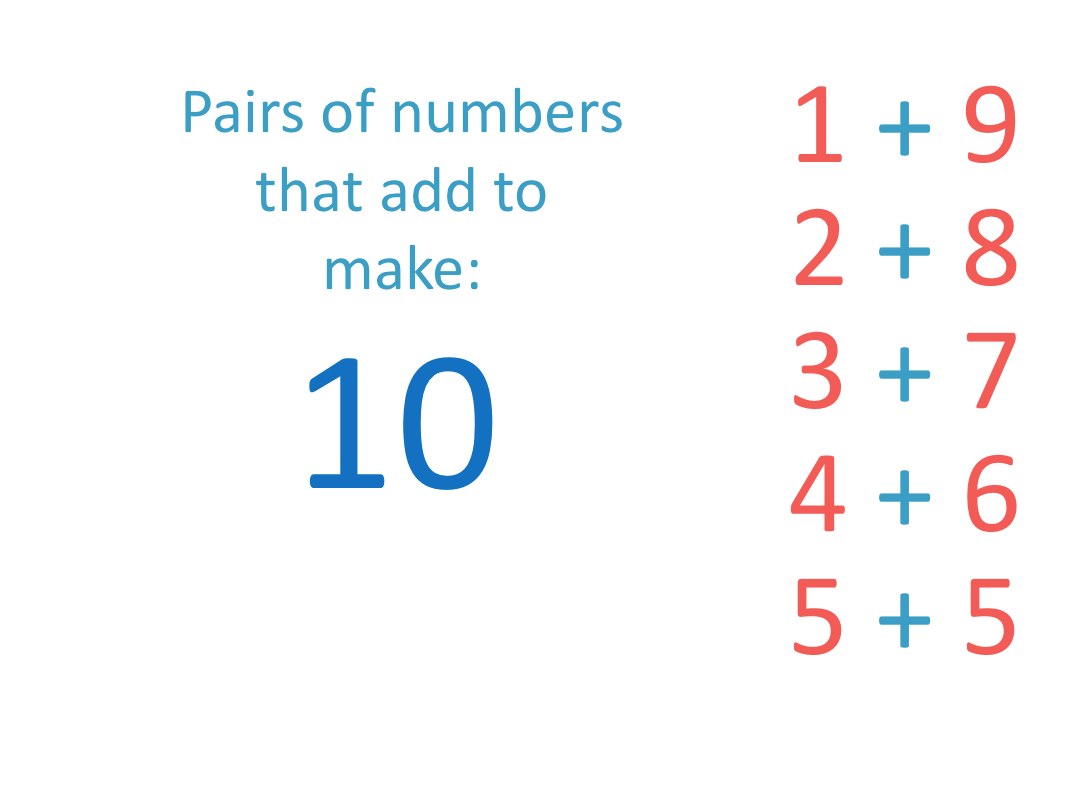Here is an example of a subtraction fact to 10, with a missing number.

We have 10 – something = 8. We need to find the missing number.Since the subtraction is from 10, we know to use the number bonds to 10 list to help us solve it.

The other number in the subtraction fact is 8.

We look at the list of number bonds to 10 and see that 2 + 8 = 10.

10 is 2 more than 8 and so if we take away 2 from 10 we will have 8.

10 – 2 = 8.

2 is our missing number.It is easier to think ‘how much larger is 10 than 8’ to solve this subtraction fact problem.

The missing number is the difference between the two numbers that are in this subtraction sentence.

Here is another subtraction fact example.

The subtraction is from 10 and so, the number bonds to 10 will help to solve it.

10 – something = 9.

This missing number is how much larger 10 is than 9.

We can see that 1 + 9 = 10 and so, 10 is 1 larger than 9.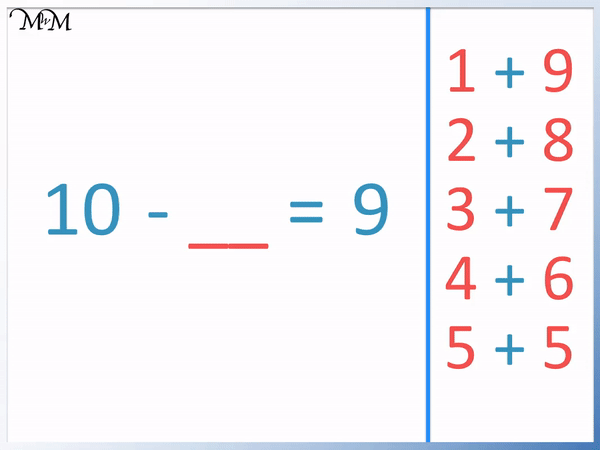The missing number is 1.To solve this subtraction from 10, we can simply look at the number bonds to 10 and see which number pairs with 9 to make 10. The missing number is this difference.

In this subtraction fact example, we want to know what 10 – 6 is equal to.

The answer is the difference between 10 and 6. It is the number that we need to add to 6 to make 10.Using the number bonds, 4 + 6 = 10 and so 4 is the missing number.Here is another subtraction from 10.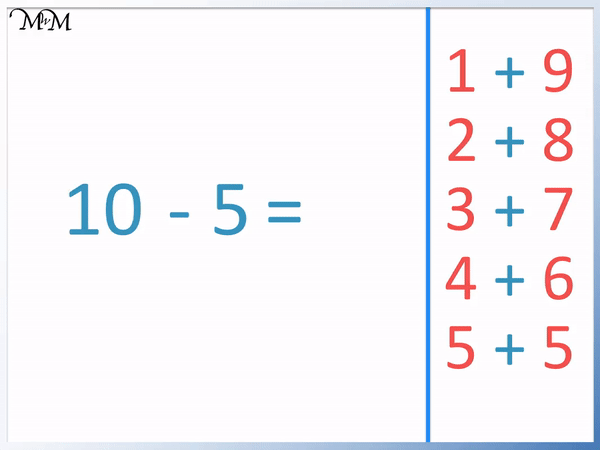5 + 5 = 10 and so, 10 – 5 = 5.When teaching subtraction from 10, it is best to try to learn the five number bonds to 10 soon after the concept has been grasped. This will help when moving on to subtracting mentally.

Here are some more subtraction from 10 questions.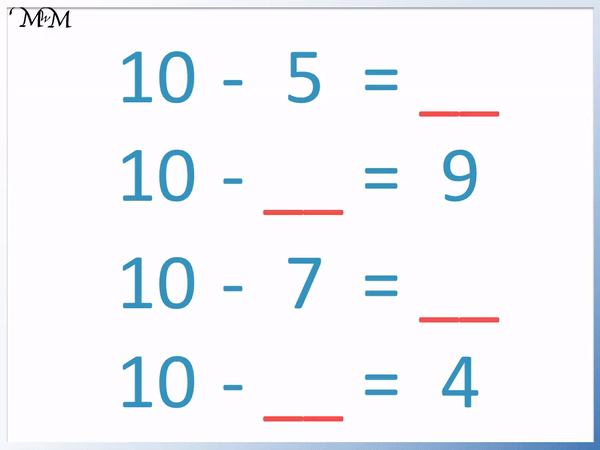## Subtraction Facts to 9

Subtraction facts to 9 are the sums made from a subtraction from 9. Because we are subtracting from 9, we use our number bonds to 9 to learn them.

Here are the number bonds to 9. The number bonds to 9 are the pairs of numbers that add to make 9.The number bonds to 9 are 1 + 8, 2 + 7, 3 + 6 and 4 + 5.Here is an example subtraction fact to 9 question. 9 – something = 3.

We are subtracting from 9 and so, we use our number bonds to 9.We see that 3 + 6 = 9 and so, 9 is 6 more than 3.

We need to subtract 6 from 9 to make 3.In this example we have a subtraction from 9 to get 7 as an answer.

The answer is the number that is subtracted from 9 to make 7.Using the number bonds to 9, 2 + 7 = 9.

And so, 9 – 2 =7.In this example we have 9 – 5.

We are subtracting from 9 and so, use our number bonds to 9 to solve this subtraction fact.

4 + 5 = 9 and so, 9 – 5 = 4.Here are some example of subtraction facts from 9 questions with their answers.## Subtraction Facts to 8

A subtraction fact from 8 is a subtraction sum in which 8 is the number being subtracted from.

To learn the subtraction facts to 8, the number bonds to 8 will be used.

The four number bonds to 8 are 1 + 7, 2 + 6, 3 + 5 and 4 + 4.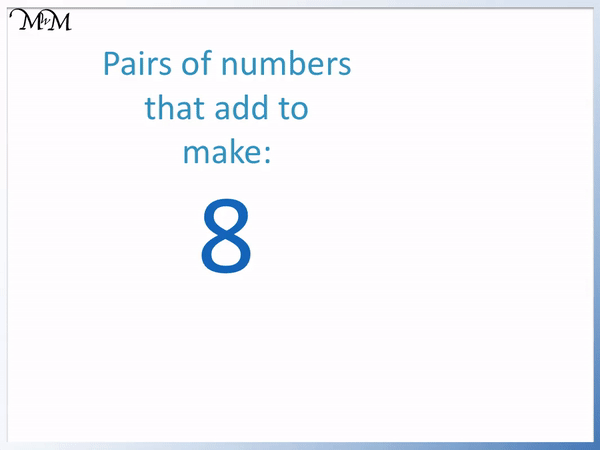In the example below we have 8 – something = 5.3 + 5 = 8 and so, 8 – 3 = 5.

In this example we have 8 – something = 4.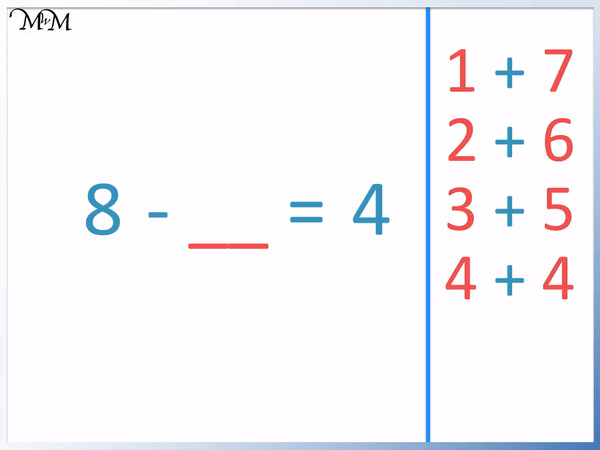4 + 4 = 8 and so, 8 is 4 more than 4.

8 – 4 = 4

In this subtraction fact to 8 question we have 8 – 6.2 + 6 = 8 and so, 8 – 6 = 2.

Here are some further subtraction fact to 8 questions and answers.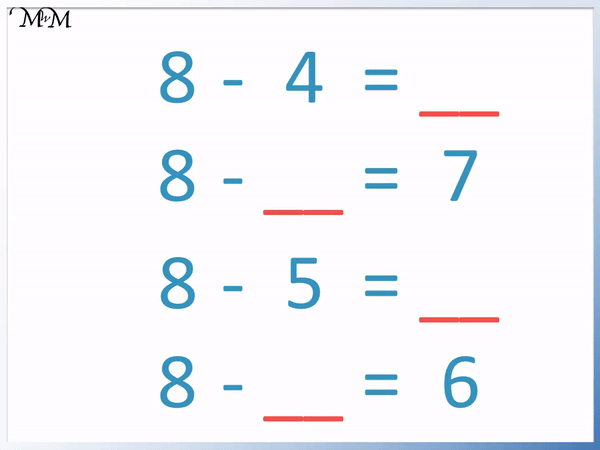## Subtraction Facts to 7

Subtraction facts to 7 are subtraction sums in which the number being subtracted from is 7.

To learn the subtraction facts to 7, we will learn the pairs of numbers that add to make 7.

Here are the number bonds to 7.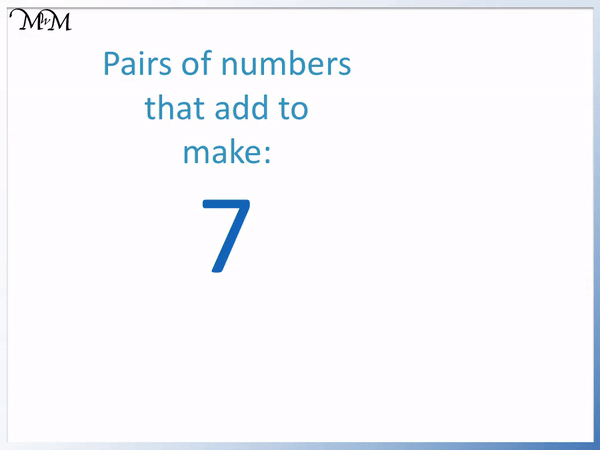The three number bonds to 7 are 1 + 6, 2 + 5 and 3 + 4.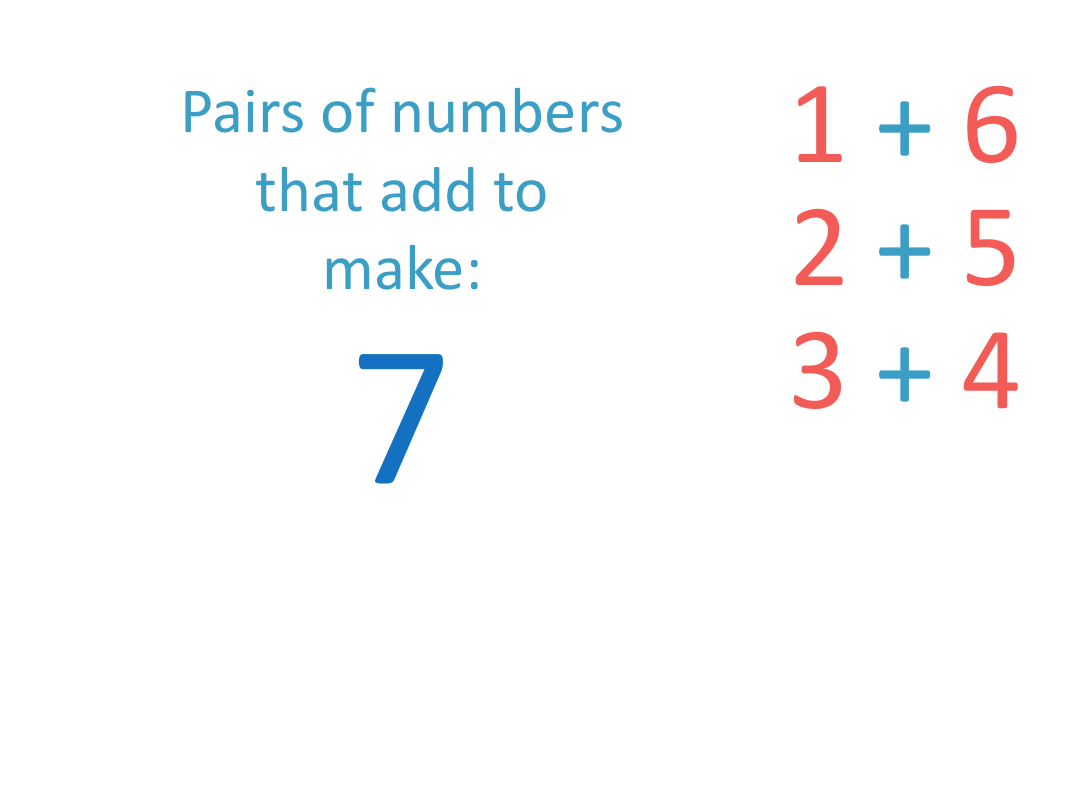In the example below we have a subtraction fact to 7 question.

7 subtract what number equals 5?Using the number bonds to 7, we know that 2 + 5 = 7.

7 is 2 more than 5 and therefore 7 subtract 2 = 5.

The missing number is 2.In this question we are asked which number is subtracted from 7 to make 3?3 + 4 = 7 and so, 7 – 4 = 3.

The missing number is 4.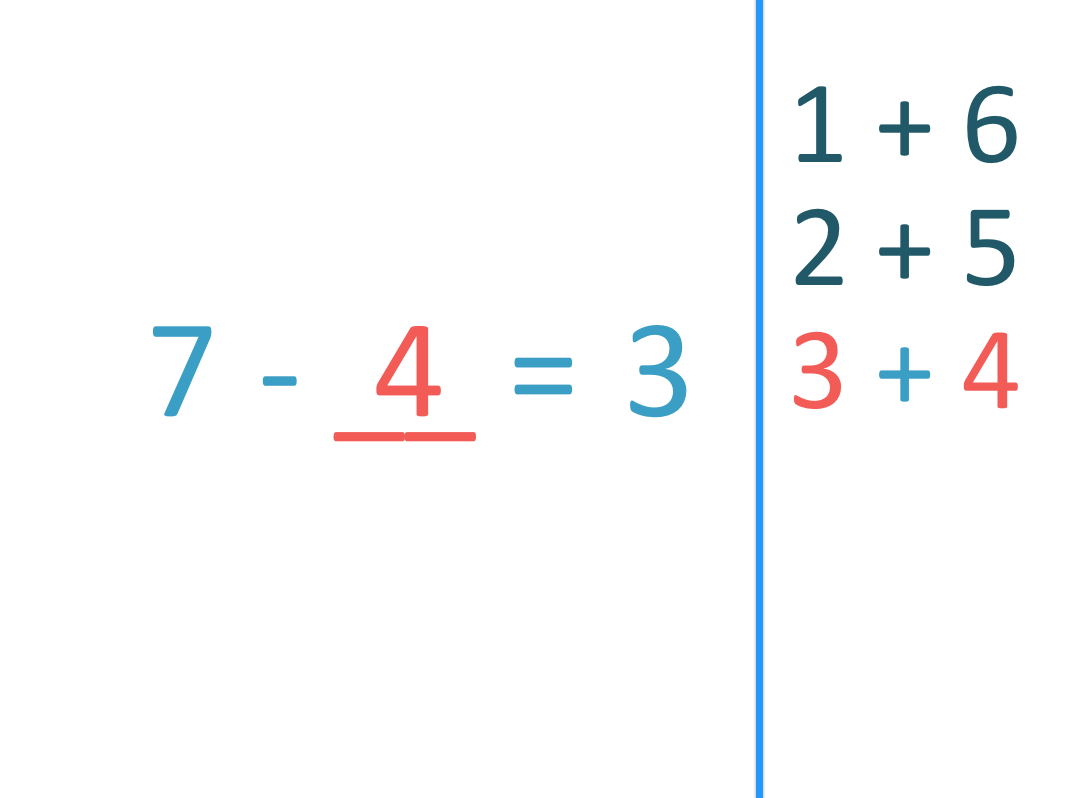Here are some subtraction fact to 7 questions with their answers.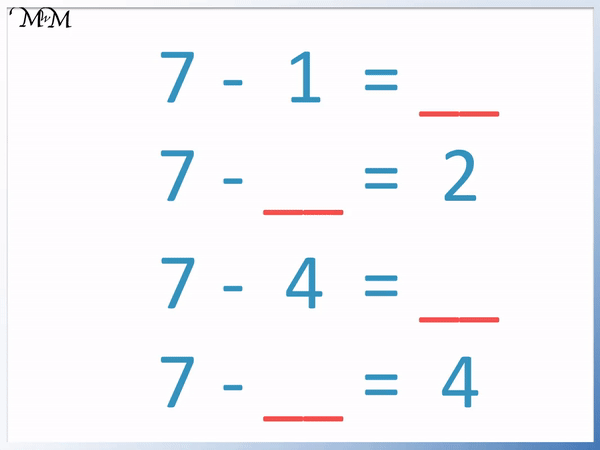Now try our lesson on Hundreds, Tens and Units where we learn how to read, write and use three-digit numbers.error: Content is protected !!